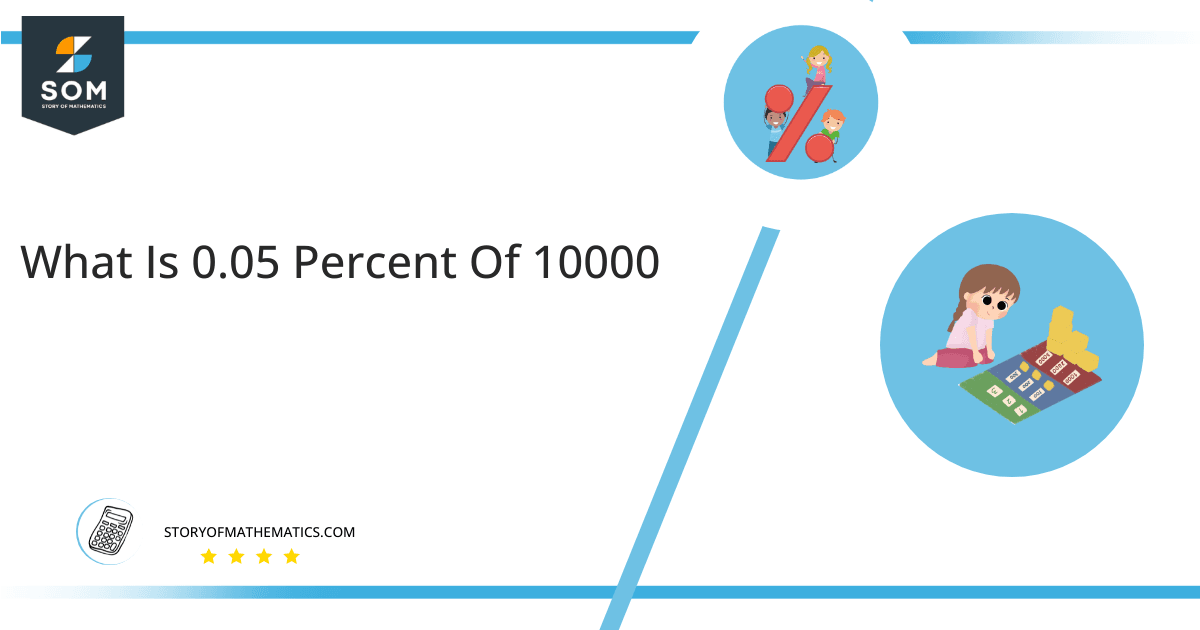# What Is 0.05 Percent of 10000 + Solution with Free Steps?The 0.05 percent of 10000 is equal to 5. It can be easily calculated by dividing 0.05 by 100 and multiplying the answer with 10000 to get 5.

The easiest way to get this answer is by solving a simple mathematical problem of percentage. You need to find 0.05% of 10000 for some sale or real-life problem. Divide 0.05 by 100, multiply the answer with 10000, and get the 0.05% of 10000 value in seconds.

This article will explain the full process of finding any percentage value from any given quantity or number with easy and simple steps.

## What Is 0.05 percent of 10000?

The 0.05 percent of 10000 is 5.

The percentage can be understood with a simple explanation. Take 10000, and divide it into 100 equal parts. The 0.05 number of parts from the total 100 parts is called 0.05 percent, which is 5 in this example.

## How To Calculate 0.05 percent of 10000?

You can find 0.05 percent of 10000 by some simple mathematical steps explained below.### Step 1

Firstly, depict 0.05 percent of 10000 as a fractional multiple as shown below:

0.05% x 10000

### Step 2

The percentage sign % means percent, equivalent to the fraction of 1/100.

Substituting this value in the above formula:

= (0.05/100) x 10000

### Step 3

Using the algebraic simplification process, we can arithmetically manipulate the above equation as follows:

= (0.05 x 10000) / 100

= 500 / 100

= 5This percentage can be represented on a pie chart for visualization. Let us suppose that the whole pie chart represents the 10000 value. Now, we find 0.05 percent of 10000, which is 5. The area occupied by the 5 value will represent the 0.05 percent of the total 10000 value. The remaining region of the pie chart will represent 99.95 percent of the total 10000 value. The 100% of 10000 will cover the whole pie chart as 10000 is the total value.

Any given number or quantity can be represented in percentages to better understand the total quantity. The percentage can be considered a quantity that divides any number into hundred equal parts for better representation of large numbers and understanding.

Percentage scaling or normalization is a very simple and convenient method of representing numbers in relative terms. Such notations find wide application in many industrial sectors where the relative proportions are used.

All the Mathematical drawings/images are created using GeoGebra.